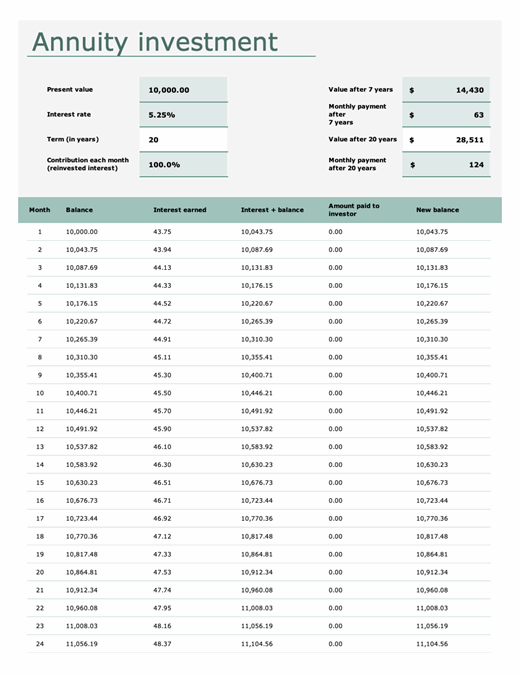Simplify the complex with data types in Excel. DOWNLOAD THE TEMPLATES NOW.

# Annuity investment calculator

This annuity calculator template shows the monthly value of an annuity investment. Simply enter the present value, interest rate, term, and contribution of reinvested interest each month, and interest and balances are calculated automatically. This annuity investment calculator includes instructions for proper use.

Excel